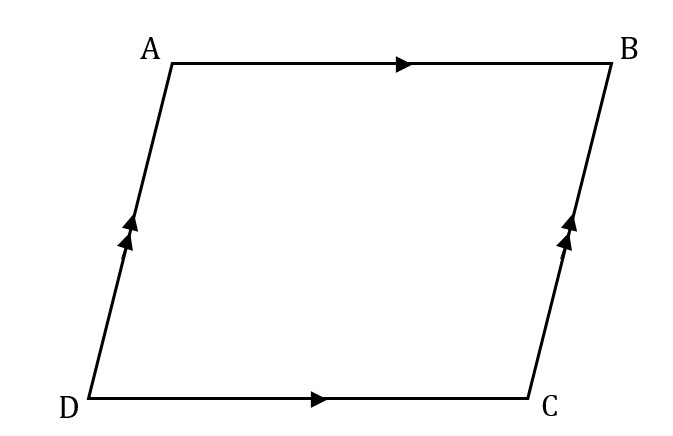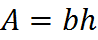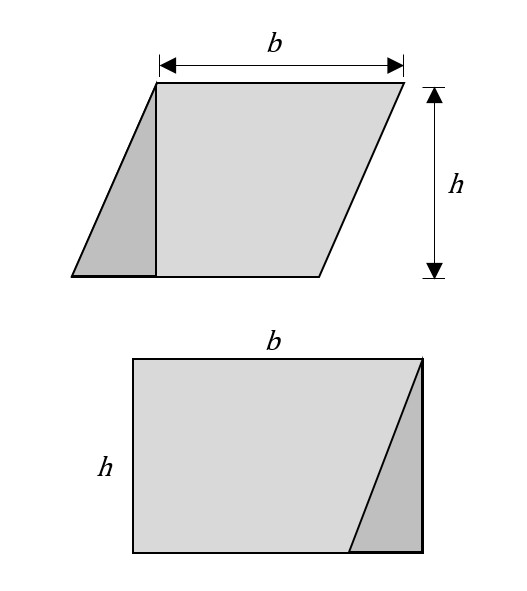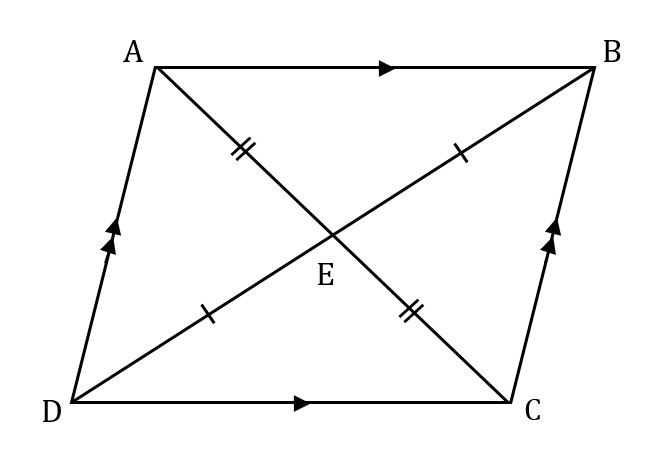# Parallelograms

A parallelogram is a quadrilateral with two pairs of parallel sides. The opposite or facing sides of a parallelogram are of equal length, and the opposite angles of a parallelogram are of equal measure.A parallelogram

## Area

The formula for the area of a parallelogram is the same as that for a rectangle’s:To make sense of this fact, notice that, as depicted below, a parallelogram with base b and height h can be divided into a trapezoid and a right triangle and rearranged into a rectangle of equivalent area.A parallelogram can be rearranged into a rectangle of the same area

## Diagonals Bisect

You should know that the diagonals of a parallelogram bisect each other. For example, in this figure,since ABCD is a parallelogram, we know that AE = EC and BE = ED, as is indicated by the single and double hash marks.

## Practice Question

Angles of a Parallelogram:
http://www.gmatfree.com/Angles-of-a-Parallelogram

Welcome! You are encouraged to register with the site and login (for free). When you register, you support the site and your question history is saved.